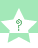Mini quiz: Answer multiple choice questions.# A Little Ditty About a Little Thing We Call The Mole

In chemistry, there is a number that is often confused with being a unit. It is not. That is the mole (mol in formulas, the formal name is Avogadro's constant). Mole is the number 6.02x10^23. If I were to say "I have 3 mole blades of grass in my lawn," that would mean I have 3x6.02x10^23 blades of grass. However, if I were to say, "I have 3 moles of sugar," that would have no meaning. I could mean grains, cubes, bags, etc. OK. Now, do you know what a molar mass is??? If you still remember it is the mass of a mole of particles; it is measured in AMU (atomic mass unit) if it is in a particle level, but it is measured in grams in mole level of particles. In our chem tool called the periodic table, we can find it as the other number other than the atomic number. Sorry, couldn't think of another way to say it. There is another concept called molar volume. This is very important to gases, but no other states of matter. The volume of one mole of gas is 22.4L/mole @ STP. Both of these can be used to find the other. If you are given, say, the molar mass, then you multiply by mole over the molar mass of the substance by 22.4 L/mole over mole.
One final concept important to understanding the behavior of gases is the formula PV=nRT (sometimes pronounced "pervnert"). In this formula, P is the pressure in kPa, V is the volume of the gas in liters, n is the number of moles, R is (.0821kPa x L)/(mol x K), and T is the temperature in Kelvin.This activity was created by a Quia Web subscriber. Learn more about QuiaCreate your own activities